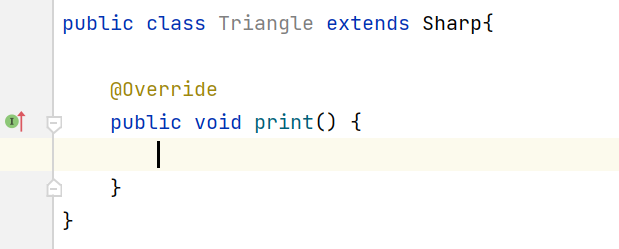﻿ Java抽象类和接口使用梳理_java_脚本之家
java# Java抽象类和接口使用梳理

## 抽象类### 抽象方法

public abstract void print();

public abstract void print();//这是没有方法体

public abstract void print(){  } //这是方法体为空，它是普通方法

### 抽象类三大原则

1.抽象类无法直接实例化对象。例如上面的 Sharp 类，Sharp  sharp  = new Sharp();//这是错误的

2.子类继承抽象类，必须覆写抽象类中的所有抽象方法（前提是子类是普通类）Triangle是一个普通类，此时没覆写方法就报错了，这时我们使用alt + enter 快捷键，点击第一行，再点击ok,就覆写了父类的抽象方法3.final 和 abstract 不能同时使用、private 和 abstract 不能同时使用abstract class Person

class Chinese extends Person  √

class Dog extends Person × //因为Dog not is a Person

## 接口

Java使用 interface 关键字定义接口，接口中只有全局常量和抽象方法（JDK之前）JDK8扩展了default方法。子类使用 implements 实现接口

public static final int NUM = 10;

public abstract String msg( );

### 接口使用原则

1.接口中只有 public 权限，且只有全局常量和抽象方法，所以 abstract、static、final、public这些关键字在接口内部都可以省略

```public interface IMassage {
int NUM = 10;//全局常量
String msg();//抽象方法
}```

2.接口没有单继承限制，子类可以同时实现多个父接口，多个接口之间使用逗号分隔。此时子类必须实现父类接口中所有的抽象方法

```public interface IMassage {
int NUM = 10;//全局常量
String msg();//抽象方法
}
interface INews{
void getNews();
}

//子类
public class MessageImpl implements IMassage,INews{

public String msg() {
return null;
}
public void getNews() {

}
}```

```    interface A{
void testA();
}
interface B{
void testB();
}
interface C extends A,B{

}```

3.接口依然不能直接实例化对象，需要通过向上转型实现

```public class MessageImpl implements IMassage,INews{

public String msg() {
return "hello JAVA";
}

public void getNews() {
System.out.println("hello n~");

}

public static void main(String[] args) {
IMassage m = new MessageImpl();
System.out.println(m.msg());

}
}
```

//输出：hello JAVA

m只能调用msg方法，不能调用INews接口类定义的方法，需要用父类间的相互转换实现调用

```        INews n = (INews)m;
n.getNews();
```

//输出：hello n~

4.子类若既要继承类又要实现接口，就先继承，后实现接口

public class D  extends A  implements X,Y{  }

### JDK两大内置接口

#### java.lang.Comparable 比较接口

```public class Student {
private String name;
private int age;
public Student(String name,int age){
this.name = name;
this.age = age;
}
public String toString(){
return "Student{" +
"name=" + name+'\''+ ",age="+ age + '}';
}

public static void main(String[] args) {
Student s1 = new Student("张三",18);
Student s2 = new Student("李四",20);
Student s3 = new Student("王五",30);
Student[] students = {s3,s1,s2};
Arrays.sort(students);
System.out.println(Arrays.toString(students));
}
}``````public class Student implements Comparable<Student>{
private String name;
private int age;
public Student(String name,int age){
this.name = name;
this.age = age;
}
public String toString(){
return "Student{" +
"name=" + name+'\''+ ",age="+ age + '}';
}
@Override
public int compareTo(Student o) {
if (this.age == o.age){
return 0;
}else if (this.age < o.age){
return -1;
}
return 1;
}

public static void main(String[] args) {
Student s1 = new Student("张三",18);
Student s2 = new Student("李四",20);
Student s3 = new Student("王五",30);
Student[] students = {s3,s1,s2};//乱序放入数组
Arrays.sort(students);
System.out.println(Arrays.toString(students));
}

}```

//输出结果：
[Student{name=张三',age=18}, Student{name=李四',age=20}, Student{name=王五',age=30}]• =0 表示当前对象等于目标对象 o
• >0 表示当前对象等于目标对象 o
• <0 表示当前对象等于目标对象 o

#### java.lang.Cloneable 克隆接口

Cloneable 接口是一个标记接口，本身没有任何抽象方法，当一个类实现了 Cloneable 接口，就表示该类具备了克隆的能力，这个能力是JVM赋予的，要知道在堆上开辟空间和对象创建都由JVM实现。```public class Cat implements Cloneable{
private String name;

@Override
protected Cat clone() throws CloneNotSupportedException {
return (Cat) super.clone();
}

public static void main(String[] args) throws CloneNotSupportedException {
Cat c1 = new Cat();
c1.name = "喵喵";
Cat c2 = c1.clone();
System.out.println(c1 == c2);
System.out.println(c2.name);
}
}```### 对象的深浅拷贝

```class A{
int num;
}
public class B implements Cloneable{
A a = new A();

@Override
protected B clone() throws CloneNotSupportedException {
return (B)super.clone();
}

public static void main(String[] args) throws CloneNotSupportedException {
B b1 = new B();
B b2 = b1.clone();
System.out.println(b1 == b2);
System.out.println(b2.a.num);
b1.a.num = 100;
System.out.println(b2.a.num);
}
}
```

0
100```class A implements Cloneable{
int num;

@Override
protected A clone() throws CloneNotSupportedException {
return (A)super.clone();
}
}
public class B implements Cloneable{
A a = new A();

@Override
protected B clone() throws CloneNotSupportedException {
B b = new B();
b.a = a.clone();
return b;
}

public static void main(String[] args) throws CloneNotSupportedException {
B b1 = new B();
B b2 = b1.clone();
System.out.println(b1 == b2);
System.out.println(b2.a.num);
b1.a.num = 100;
System.out.println(b2.a.num);
}
}
```

0
0

## 抽象类和接口的区别